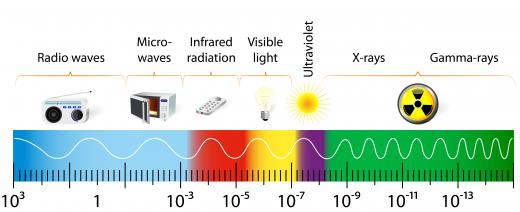# What is Electromagnetic Wavelength?

John Kinsellagh
John KinsellaghThe full range of electromagnetic waves is identified as the electromagnetic spectrum.

The phenomenon of electromagnetic radiation is caused by the mutually reinforcing interaction of charged electrical and magnetic fields operating perpendicular to each other and that travel through space at the speed of light. Each pulse or oscillation emanating from the interplay of the electrical and magnetic force fields creates a wave of energy. Electromagnetic wavelength refers to the measured distance between the crest or trough of each adjacent wave generated by the electromagnetic disturbance. People experience the various forms of electromagnetic radiation frequently in their everyday lives. Radio waves, television broadcasts, X-rays, visible and invisible light, and microwave radiation are each discrete components of the electromagnetic spectrum that can be defined and categorized by their respective electromagnetic wavelengths.

Scottish physicist James Clerk Maxwell first developed the theory of electromagnetism in the 19th century. Maxwell observed that the changes in an electric field caused magnetic force fields, which in turn induced electrical fields. Maxwell predicted that these mutually reinforcing force fields would interact with each other at right angles in a plane, creating oscillations that would propagate thorough space at the speed of light.Physicist James Clerk Maxwell came up with the theory of electromagnetism.

Since all forms of electromagnetic radiation consist of waves of energy traveling through space, electromagnetic wavelength is one of the principal measures that is used to classify the discrete components of the entire electromagnetic spectrum. At the long wave end of the spectrum are radio transmissions, whose measured electromagnetic wavelengths can be the size of buildings. At the opposite end of the spectrum are gamma rays, whose wavelengths are smaller than the size of an atom's nucleus. Arrayed between long wavelength radio transmissions and ultra short electromagnetic wavelength gamma rays, in order of increasing wavelength, are microwaves, infrared radiation, visible light, ultra violet light, and X-rays.

The intensity of electromagnetic radiation generated is a function of the frequency of the waves generated each second. The incident of each complete wave constitutes a cycle. Specific frequencies are identified by the number of cycles generated each second. The international unit used to measure each complete cycle is one Hertz, or in its abbreviated form, Hz.

Both the frequency and wavelength of electromagnetic radiation are mathematically related. The energy of the electromagnetic radiation generated is directly proportional to its frequency. The higher the frequency, the greater the propagated radiation. Conversely, the frequency and wavelength of electromagnetic radiation are inversely related; the higher the frequency of radiation generated, the lower the electromagnetic wavelength and vice versa.